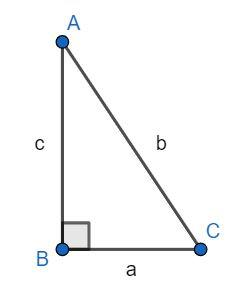QUESTION

# Evaluate the given trigonometric ratio $\dfrac{{\sin 18^\circ }}{{\cos 72^\circ }}$.

Hint: Recall the properties of trigonometric and the relations between them. Use the property given by $\sin (90^\circ - x) = \cos x$ to simplify the expression.

A function of an angle expressed as the ratio of two of the sides of a right triangle that contains that angle is called trigonometric functions.

The sine, cosine, tangent, cotangent, secant, and cosecant are the trigonometric functions.
The sine and cosecant are inverses of each other. The cosine and secant are inverses of each other. The tangent and the cotangent are inverses of each other.

They all are related to one another with special formulas.

Consider a right-angled triangle ABC, right-angled at B as shown in the diagram.The sum of the angles of the triangle is 180°. Hence, we have:

$A + B + C = 180^\circ$

We know that B is a right angle, hence, we have:

$A + 90^\circ + C = 180^\circ$

Finding angle A in terms of C, we get:

$A = 180^\circ - 90^\circ - C$

Simplifying, we get:

$A = 90^\circ - C..............(1)$

The sine of an angle is the ratio of the opposite side to the hypotenuse side, hence, the value of the sine of angle C is given by:

$\sin C = \dfrac{c}{a}...........(2)$

Substituting equation (1) in equation (2), we get:

$\sin (90^\circ - A) = \dfrac{c}{a}...........(3)$

The cosine of an angle is the ratio of the adjacent side to hypotenuse side, hence, the value of the cosine of angle A is given by:

$\cos A = \dfrac{c}{a}...........(4)$

Equating equation (3) and equation (4), we have:

$\sin (90^\circ - A) = \cos A............(5)$

Now, we use the property in equation (5) to evaluate $\dfrac{{\sin 18^\circ }}{{\cos 72^\circ }}$ as follows:

$\dfrac{{\sin 18^\circ }}{{\cos 72^\circ }} = \dfrac{{\sin 18^\circ }}{{\sin (90^\circ - 72^\circ )}}$

Simplifying, we get:

$\dfrac{{\sin 18^\circ }}{{\cos 72^\circ }} = \dfrac{{\sin 18^\circ }}{{\sin (18^\circ )}}$

Canceling the terms, we have:

$\dfrac{{\sin 18^\circ }}{{\cos 72^\circ }} = 1$

Hence, the value of the given expression is 1.

Note: You can also use the formula $\sin A = \cos (90^\circ - A)$ to evaluate the expression and simplify to get the final answer. The final answer is invariant of what substitution you use.